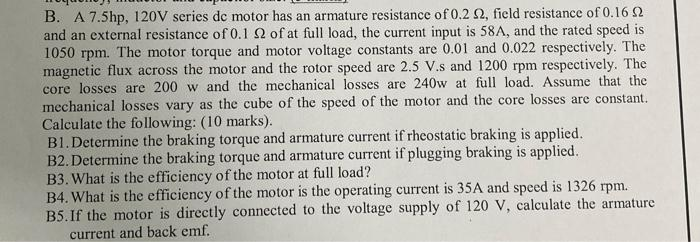# Question Solved1 AnswerB. A $$7.5 \mathrm{hp}, 120 \mathrm{~V}$$ series dc motor has an armature resistance of $$0.2 \Omega$$, field resistance of $$0.16 \Omega$$ and an external resistance of $$0.1 \Omega$$ of at full load, the current input is $$58 \mathrm{~A}$$, and the rated speed is $$1050 \mathrm{rpm}$$. The motor torque and motor voltage constants are $$0.01$$ B. A 7.5hp,120 V series dc motor has an armature resistance of 0.2Ω, field resistance of 0.16Ω and an external resistance of 0.1Ω of at full load, the current input is 58 A, and the rated speed is 1050rpm. The motor torque and motor voltage constants are 0.01 and 0.022 respectively. The magnetic flux across the motor and the rotor speed are 2.5 V.s and 1200rpm respectively. The core losses are 200w and the mechanical losses are 240w at full load. Assume that the mechanical losses vary as the cube of the speed of the motor and the core losses are constant. Calculate the following: (10 marks). B1. Determine the braking torque and armature current if rheostatic braking is applied. B2. Determine the braking torque and armature current if plugging braking is applied. B3. What is the efficiency of the motor at full load? B4. What is the efficiency of the motor is the operating current is 35 A and speed is 1326rpm. B5. If the motor is directly connected to the voltage supply of 120 V, calculate the armature current and back emf.1WGQX5 The Asker · Mechanical EngineeringTranscribed Image Text: B. A 7.5hp,120 V series dc motor has an armature resistance of 0.2Ω, field resistance of 0.16Ω and an external resistance of 0.1Ω of at full load, the current input is 58 A, and the rated speed is 1050rpm. The motor torque and motor voltage constants are 0.01 and 0.022 respectively. The magnetic flux across the motor and the rotor speed are 2.5 V.s and 1200rpm respectively. The core losses are 200w and the mechanical losses are 240w at full load. Assume that the mechanical losses vary as the cube of the speed of the motor and the core losses are constant. Calculate the following: (10 marks). B1. Determine the braking torque and armature current if rheostatic braking is applied. B2. Determine the braking torque and armature current if plugging braking is applied. B3. What is the efficiency of the motor at full load? B4. What is the efficiency of the motor is the operating current is 35 A and speed is 1326rpm. B5. If the motor is directly connected to the voltage supply of 120 V, calculate the armature current and back emf.
More
Transcribed Image Text: B. A 7.5hp,120 V series dc motor has an armature resistance of 0.2Ω, field resistance of 0.16Ω and an external resistance of 0.1Ω of at full load, the current input is 58 A, and the rated speed is 1050rpm. The motor torque and motor voltage constants are 0.01 and 0.022 respectively. The magnetic flux across the motor and the rotor speed are 2.5 V.s and 1200rpm respectively. The core losses are 200w and the mechanical losses are 240w at full load. Assume that the mechanical losses vary as the cube of the speed of the motor and the core losses are constant. Calculate the following: (10 marks). B1. Determine the braking torque and armature current if rheostatic braking is applied. B2. Determine the braking torque and armature current if plugging braking is applied. B3. What is the efficiency of the motor at full load? B4. What is the efficiency of the motor is the operating current is 35 A and speed is 1326rpm. B5. If the motor is directly connected to the voltage supply of 120 V, calculate the armature current and back emf.